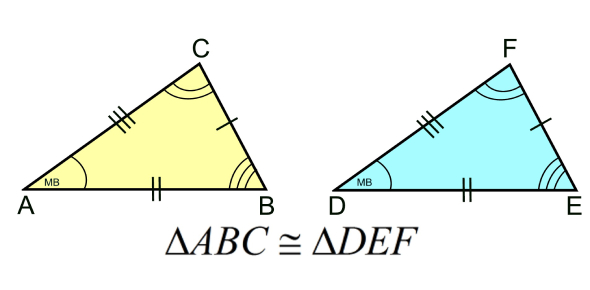# A Test On Triangles! Math Trivia Quiz

15 Questions | Attempts: 2441Settings.

• 1.
• A.

XY = QR

• B.

XY = 1/3 QR

• C.

XY2 = QR2

• D.

XY = ½ QR

• 2.
In the following figure QA⊥AB and PB⊥Ab, then AQ is:
• A.

15 units

• B.

8 units

• C.

5 units

• D.

9 units

• 3.
The ratio of the areas of two similar triangles is equal to the:
• A.

Ratio of their corresponding sides

• B.

Ratio of their corresponding altitudes

• C.

Ratio of the squares of their corresponding sides

• D.

Ratio of the squares of their perimeter

• 4.
The areas of two similar triangles are 144 cm2 and 81 cm2. If one median of the first triangle is 16 cm, length of corresponding median of the second triangle is:
• A.

9 cm

• B.

27 cm

• C.

12 cm

• D.

16 cm

• 5.
• A.

13 units

• B.

12 units

• C.

6 units

• D.

15 units

• 6.
If ∆ ABC ~ ∆ DEF, are (∆ DEF) 100 cm2 and AB/DE = ½ then ar (∆ABC) is:
• A.

50 cm2

• B.

25 cm2

• C.

4 cm2

• D.

None of the above

• 7.
• A.

450

• B.

300

• C.

600

• D.

900

• 8.
If ∠ACB = 900 and CD ⊥ AB then:
• A.
• B.
• C.
• D.
• 9.
If XY ∥ AC of ΔABC and it divides the triangle into two parts of equal areas. Then AX/AB is:
• A.
• B.
• C.
• D.
• 10.
A girl of height 80 cm is running away from the base of a lamp-post at a speed of 2 m/s. If the lamp is 4 m above the ground, find the length of her shadow after 5 seconds.
• A.

1.6 m

• B.

2.5 m

• C.

2 m

• D.

3 m

• 11.
• A.

An equilateral triangle

• B.

An isosceles triangle

• C.

A right triangle

• D.

None of the above

• 12.
If in two triangles, sides of one triangle are proportional to the side of other triangle. Then:
• A.

The alternate angles are equal

• B.

The vertically opposite angle are equal

• C.

The corresponding angles are equal

• D.

None of the above

• 13.
If a line divides any two sides of a triangle in the same ratio, then:
• A.

The line equal to the third line

• B.

The line is perpendicular to the third line

• C.

The line is parallel to the third line

• D.

The line is equal to the sum of two sides of the triangle

• 14.
BL and CM are medians of a triangle ABC right angled at A. Then 4(BL2 + CM2) is:
• A.

5BC2

• B.

3BC2

• C.

3LM2

• D.

5LM2

• 15.
A ladder is placed against a wall such that its foot is at a distance of 5 m from the wall and its top reaches a window 12 m above the ground. Find the length of the ladder.
• A.

11 m

• B.

12.5 m

• C.

11.5 m

• D.

13 m

## Related TopicsBack to top
×

Wait!
Here's an interesting quiz for you.